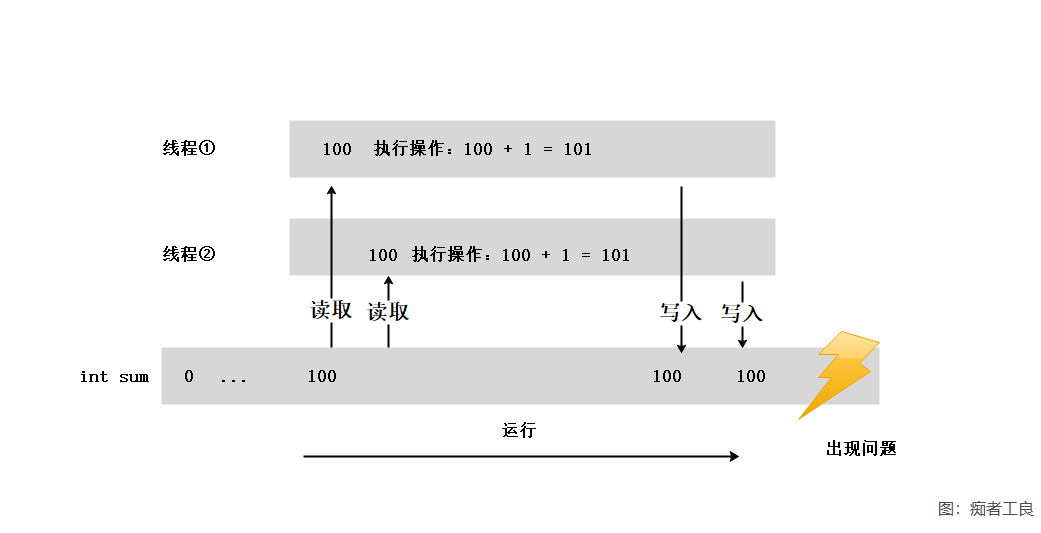# C#多线程系列(3)：原子操作

## 知识点

### 内核模式和用户模式

`Sleep()``Join()` 等，都是使用内核模式来阻塞线程，实现线程同步(等待)。

## Interlocked类

Interlocked 类是静态类，让我们先来看看 Interlocked 的常用方法：

CompareExchange() 比较两个数是否相等，如果相等，则替换第一个值。
Decrement() 以原子操作的形式递减指定变量的值并存储结果。
Exchange() 以原子操作的形式，设置为指定的值并返回原始值。
Increment() 以原子操作的形式递增指定变量的值并存储结果。

### 1,出现问题

​ C# 中赋值和一些简单的数学运算不是原子操作，受多线程环境影响，可能会出现问题。

``````        private static int sum = 0;
{
for (int i = 0; i < 100_0000; i++)
{
sum += 1;
}
}``````

``````        static void Main(string[] args)
{
Console.WriteLine("sum = " + sum);
}``````

``````        static void Main(string[] args)
{
for (int i = 0; i < 5; i++)
{
}

Console.WriteLine("sum = " + sum);
}``````

``````8757
8758
8760
8760
8760
8761
8762
8763
8764
8765
8766
8766
8768
8769``````### 2,Interlocked.Increment

``````        public static void AddOne()
{
for (int i = 0; i < 100_0000; i++)
{
Interlocked.Increment(ref sum);
}
}``````

### 3,Interlocked.Exchange

`Interlocked.Exchange()` 实现赋值运算。

``public static int Exchange(ref int location1, int value);``

``````        static void Main(string[] args)
{
int a = 1;
int b = 5;

// a 改变前为1
int result1 = Interlocked.Exchange(ref a, 2);

Console.WriteLine(\$"a新的值 a = {a}   |  a改变前的值 result1 = {result1}");

Console.WriteLine();

// a 改变前为 2，b 为 5
int result2 = Interlocked.Exchange(ref a, b);

Console.WriteLine(\$"a新的值 a = {a}   | b不会变化的  b = {b}   |   a 之前的值  result2 = {result2}");
}``````

``Exchange(T, T)``

### 4,Interlocked.CompareExchange

``public static int CompareExchange (ref int location1, int value, int comparand)``

``````        static void Main(string[] args)
{
int location1 = 1;
int value = 2;
int comparand = 3;

Console.WriteLine("运行前：");
Console.WriteLine(\$" location1 = {location1}    |   value = {value} |   comparand = {comparand}");

Console.WriteLine("当 location1 != comparand 时");
int result = Interlocked.CompareExchange(ref location1, value, comparand);
Console.WriteLine(\$" location1 = {location1} | value = {value} |  comparand = {comparand} |  location1 改变前的值  {result}");

Console.WriteLine("当 location1 == comparand 时");
comparand = 1;
result = Interlocked.CompareExchange(ref location1, value, comparand);
Console.WriteLine(\$" location1 = {location1} | value = {value} |  comparand = {comparand} |  location1 改变前的值  {result}");
}``````

``public static int Add (ref int location1, int value);``

``````        static void Main(string[] args)
{
for (int i = 0; i < 5; i++)
{
}

Console.WriteLine("sum = " + sum);
}
private static int sum = 0;
{
for (int i = 0; i < 100_0000; i++)
{
}
}``````

``public static long Read (ref long location);``